Updating search results...

# 54 Results

View
Selected filters:
• cl8lesson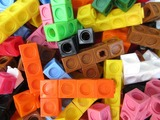Conditional Remix & Share Permitted
CC BY-NC-SA
Rating
0.0 stars

In this lesson students explore symmetry in various shapes.&nbsp;

Subject:
Mathematics
Material Type:
Activity/Lab
Lesson Plan
Author:
Drew Polly
07/25/2020Conditional Remix & Share Permitted
CC BY-NC-SA
Rating
0.0 stars

In this lesson students explore lines and angles in the context of North Carolina.&nbsp;

Subject:
Mathematics
Material Type:
Activity/Lab
Lesson Plan
Author:
Drew Polly
07/25/2020Conditional Remix & Share Permitted
CC BY-NC-SA
Rating
0.0 stars

In this mini lesson students will explore length, weight, and capacity.

Subject:
Mathematics
Material Type:
Activity/Lab
Lesson Plan
Author:
Drew Polly
07/13/2020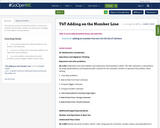Conditional Remix & Share Permitted
CC BY-NC-SA
Rating
0.0 stars

This resource is part of Tools4NCTeachers.

In this lesson students use the open number line and various strategies to solve addition story problems.

Subject:
Mathematics
Material Type:
Lesson
Lesson Plan
11/06/2019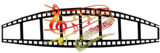Unrestricted Use
CC BY
Rating
0.0 stars

This resource is from Tools4NCTeachers.&nbsp;&nbsp;In this lesson, students determine whether or not they have enough money to purchase popcorn in addition to movie tickets. They then determine whether or not there will be change and if so, how much change they would receive. Printable student sheets are provided in this lesson.

Subject:
Mathematics
Material Type:
Activity/Lab
Lesson
Lesson Plan
Author:
DAWNE COKER
06/23/2020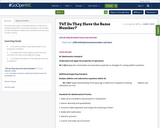Conditional Remix & Share Permitted
CC BY-NC-SA
Rating
0.0 stars

This resource is part of Tools4NCTeachers.

In this lesson, students explore the communitive property in the context of a word problem to determine if students have the same number of items

Subject:
Mathematics
Material Type:
Lesson
Lesson Plan
11/06/2019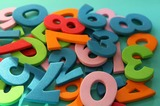Unrestricted Use
CC BY
Rating
0.0 stars

This resource is from Tools4NCTeachers.In this lesson, students explore the communitive property in the context of a word problem to determine if students have the same number of items.&nbsp; This file includes the lesson plan, student recording sheets, and extension materials/activities.

Subject:
Mathematics
Material Type:
Lesson
Lesson Plan
Author:
DAWNE COKER
06/14/2020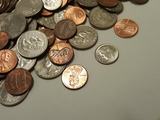Unrestricted Use
CC BY
Rating
0.0 stars

This resource is from Tools4NCTeachers.This lesson is an extension of NC.1.MD.5 to provide rigor to students who need it.&nbsp; In this lesson, students use patterns to count sets of like coins (pennies, nickels, and dimes).&nbsp; Students will connect counting patterns to counting coins and will use coins in different ways to represent the same values.&nbsp; Students will also explain their strategies for counting sets of pennies, nickels, and dimes.

Subject:
Mathematics
Material Type:
Activity/Lab
Lesson
Lesson Plan
Author:
DAWNE COKER
06/14/2020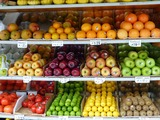Unrestricted Use
CC BY
Rating
0.0 stars

This resource is from Tools4NCTeachers.In this lesson, students explore the associative properties as they solve word problems with three addends to develop the concept of representing and solving problems involving addition.&nbsp; This lesson format may be used with any problem type appropriate for the grade.&nbsp; This file includes the lesson, student work samples, student recording sheets, and exit tickets.&nbsp; Remix this lesson to add extension ideas or addtional student work samples.&nbsp;

Subject:
Mathematics
Material Type:
Lesson
Lesson Plan
Author:
DAWNE COKER
06/14/2020Unrestricted Use
CC BY
Rating
0.0 stars

This lesson is part of Tools4NCTeachers.In this lesson, students practice solving unknown-addend problems by using addition strategies or changing to subtraction problems. Task cards and extension actvities are included.&nbsp;

Subject:
Mathematics
Material Type:
Activity/Lab
Lesson
Lesson Plan
Author:
DAWNE COKER
06/14/2020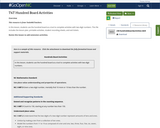Unrestricted Use
CC BY
Rating
0.0 stars

This resource is from Tools4NCTeachers.In this lesson, students use the hundred board as a tool to complete activities with two-digit numbers. This file includes the lesson plan, printable activities, student recording sheets, and exit tickets.Remix this lesson to add extension activities.

Subject:
Mathematics
Material Type:
Lesson
Lesson Plan
Author:
DAWNE COKER
06/14/2020Unrestricted Use
CC BY
Rating
0.0 stars

This resource is from Tools4NCTeachers.In this lesson, students observe and describe the characteristics of a quarter, dime, nickel, and penny to help them identify and sort the coins.&nbsp; Students will also represent the number of each coin on a bar graph and will ask and answer questions to interpret the data.&nbsp; Student materials and photos of student work are included.Remix this lesson to include extension ideas or additional pictures of student work.

Subject:
Mathematics
Material Type:
Lesson
Lesson Plan
Author:
DAWNE COKER
06/14/2020Unrestricted Use
CC BY
Rating
0.0 stars

This resource is from Tools4NCTeachers.In this lesson, students work with different quantities to determine if they have the same value / equal.&nbsp; The information is presented in a context, not simply in an equation format, so students will need to apply their understanding of problem solving and resulting equations along with computation in order to determine if the amounts are equal. &nbsp;Students will explain and defend their answers.&nbsp; This file includes lesson, student recording sheets, and printable exit tickets.&nbsp; Remix this lesson to include extension ideas or student work samples.

Subject:
Mathematics
Material Type:
Lesson
Lesson Plan
Author:
DAWNE COKER
06/14/2020Unrestricted Use
CC BY
Rating
0.0 stars

This resource is from Tools4NCTeachers.&nbsp;&nbsp;In this game, students toss pennies and dimes onto a target on the floor.&nbsp; After tossing their coins, they determine the total value of the coins landing on their target.&nbsp; The student with the greater amount wins.

Subject:
Mathematics
Material Type:
Activity/Lab
Game
Lesson
Lesson Plan
Author:
DAWNE COKER
06/23/2020Unrestricted Use
CC BY
Rating
0.0 stars

This resource is from Tools4NCTeachers.&nbsp;&nbsp;In this lesson, students solve word problems by adding and subtracting amounts up to 99 cents.&nbsp; Student recording sheets are provided.Remix this lesson to include additional word problems.

Subject:
Mathematics
Material Type:
Activity/Lab
Lesson
Lesson Plan
Author:
DAWNE COKER
06/23/2020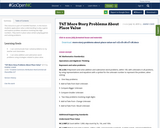Conditional Remix & Share Permitted
CC BY-NC-SA
Rating
0.0 stars

This resource is part of Tools4NCTeachers.

In this lesson students apply place value understanding to play a game and explore problem situations involving two-digit numbers. They work in place value centers playing games and solving problems.

Subject:
Mathematics
Material Type:
Lesson
Lesson Plan
11/06/2019Unrestricted Use
CC BY
Rating
0.0 stars

This resource is from Tools4NCTeachers.&nbsp;&nbsp;In this lesson students explore arrays through the context of flats of flowers.&nbsp; Students determine the total number of flowers and write an equation using equal addends.&nbsp; Students calculate the total cost of flats of flowers within 99&cent; and represent cost with variations of quarters, dimes, nickels, and pennies.

Subject:
Mathematics
Material Type:
Activity/Lab
Lesson
Lesson Plan
Author:
DAWNE COKER
06/23/2020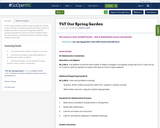Conditional Remix & Share Permitted
CC BY-NC-SA
Rating
0.0 stars

In this lesson students explore arrays through the context of flats of flowers. Students determine the total number of flowers and write an equation using equal addends. Students calculate the total cost of flats of flowers within 99¢ and represent cost with variations of quarters, dimes, nickels, and pennies.

Subject:
Mathematics
Material Type:
Lesson
Lesson Plan
11/06/2019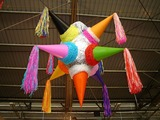Unrestricted Use
CC BY
Rating
0.0 stars

This resource is from Tools4NCTeachers.In this lesson, students will solve higher-level word problems involving three addends.&nbsp; The rich problems allow for multiple solutions, so the focus will be on student thinking rather than correct answers.&nbsp; Students will explore various strategies to solve and will be asked to attempt new strategies, including writing matching equations, once that has been modeled by others.&nbsp; &nbsp;This resource includes a lesson plan, answer key, and printable exit ticket.&nbsp; Remix this lesson to include extension activities of photos of student work samples.

Subject:
Mathematics
Material Type:
Lesson
Lesson Plan
Author:
DAWNE COKER
06/14/2020Unrestricted Use
CC BY
Rating
0.0 stars

This resource is from Tools4NCTeachers.In this lesson, students represent and interpret three categories of data in charts and tables.&nbsp; They analyze the data to answer compare questions about how many more are in one category than another.&nbsp; The lesson may be modified to integrate or emphasize questions about how many fewer.&nbsp; Samples of student work and student recording sheets are included.Remix this lesson to include extension activites or related math stations.&nbsp; Sharing is caring!

Subject:
Mathematics
Material Type:
Lesson
Lesson Plan
Author:
DAWNE COKER